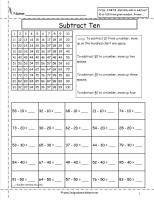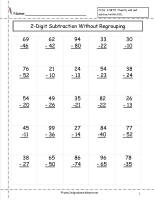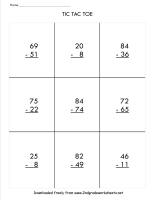# Two Digit Subtraction With and Without Regrouping Worksheets

## Two Digit Subtraction With Regrouping, Two Digit Subtraction Without Regrouping

###Count Back to Subtract Ones and Tens Worksheet

Common Core State Standards: 2.NBT.5 Fluently add and subtract within 100….
Count back to subtract ones and tens from two digit numbers.

###Subtracting Tens Worksheet

Common Core State Standards: 2.NBT.5 Fluently add and subtract within 100….
Count back to subtract tens from two digit numbers.  Hundred chart included.  25 problems

###Subtract Tens from Tens Worksheet.

Common Core State Standards: 2.NBT.5 Fluently add and subtract within 100….
Count back to subtract tens from tens.  25 problems.

###Subtract Tens from Two Digit Numbers Worksheet

Common Core State Standards: 2.NBT.5 Fluently add and subtract within 100….
Count back to subtract tens from two digit numbers.  25 problems.

###Subtracting One Digit Numbers from Two Digit Numbers Worksheet 2, 3

Common Core State Standards: 2.NBT.5 Fluently add and subtract within 100….
Subtract one digit numbers from two digit numbers.  25 problems.

###Two Digit Subtraction Without Regrouping Worksheet 2, 3,    4

Common Core State Standards: 2.NBT.5 Fluently add and subtract within 100….
Subtract two digit numbers from two digit numbers without regrouping.

###Two Digit Subtraction With Regrouping Worksheet 2, 3, 4

Common Core State Standards: 2.NBT.5 Fluently add and subtract within 100….
Subtract two digit numbers from two digit numbers with regrouping.

###Two Digit Subtraction With Regrouping Tic Tac Toe

Common Core State Standards: 2.NBT.5 Fluently add and subtract within 100….
Subtract two digit numbers from two digit numbers tic tac toe
.

###Mixed Subtraction Review

Common Core State Standards: 2.NBT.5 Fluently add and subtract within 100….
Mixed subtraction review.